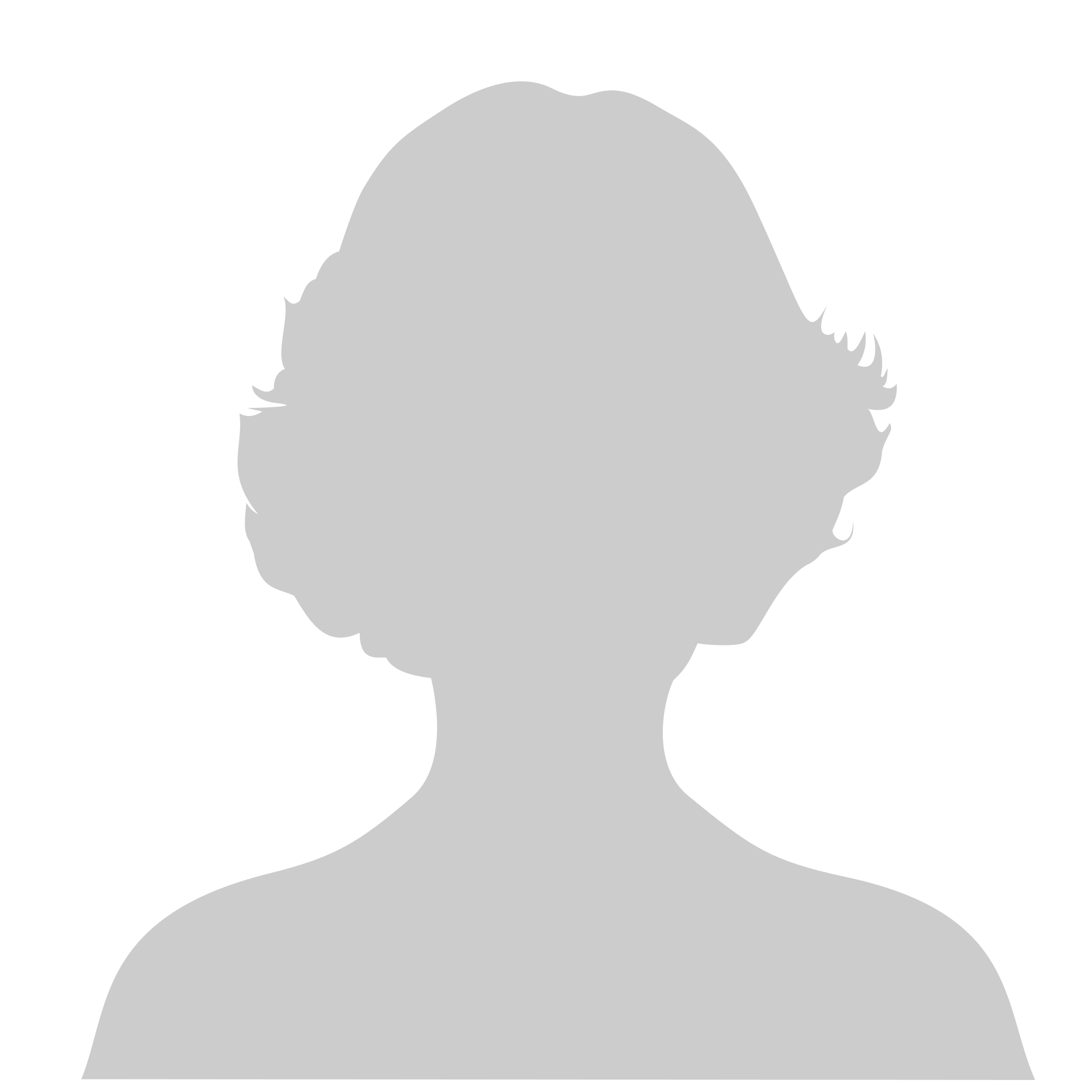# MAP 2302 – Differential Equations

General Education Credit: Mathematics
College Credit: 3Staff

Pre-Requisites: MAC 2312 or MAC 2512 or MAC 3473 with a minimum grade of C.

This course presents techniques for solving and approximating solutions to ordinary differential equations.

Course Objectives: This course is designed to serve students in engineering, physics, mathematics, and related disciplines with the goal of understanding qualitatively, applying, and solving ordinary differential equations of the most usual types.

### Course Goals

After completing the course, students should be able to:

• identify different types of ordinary differential equations (linear, nonlinear, separable, linear, exact, homogeneous, Bernoulli),
• plot the direction field of a differential equation and use it to describe properties of solutions to
• initial value problems,
• use Euler’s method to find numerical solutions to initial value problems,
• solve various types of first-order differential equations (separable, linear, exact, homogeneous,Bernoulli), initial value problems, and applications,
• provide the mathematical description of vibrating mechanical systems such as the mass-spring oscillator,
• use various methods to find general solutions and solve initial value problems of linear homogeneous and non-homogeneous second-order differential equations,
• compute the Laplace and inverse transforms of various functions,
• solve initial value problems and applications using the method of Laplace transforms,
• use the Convolution Theorem to find the Inverse Laplace transform,
• solve integral and integro-differential equations,
• find the transfer and the impulse response function for a differential equation,
• solve symbolic initial value problems,
• use some power series methods to solve ODEs and initial value problems.

Satisfied High School Graduation Requirement Subject: Mathematics
High School Credit: 1.0